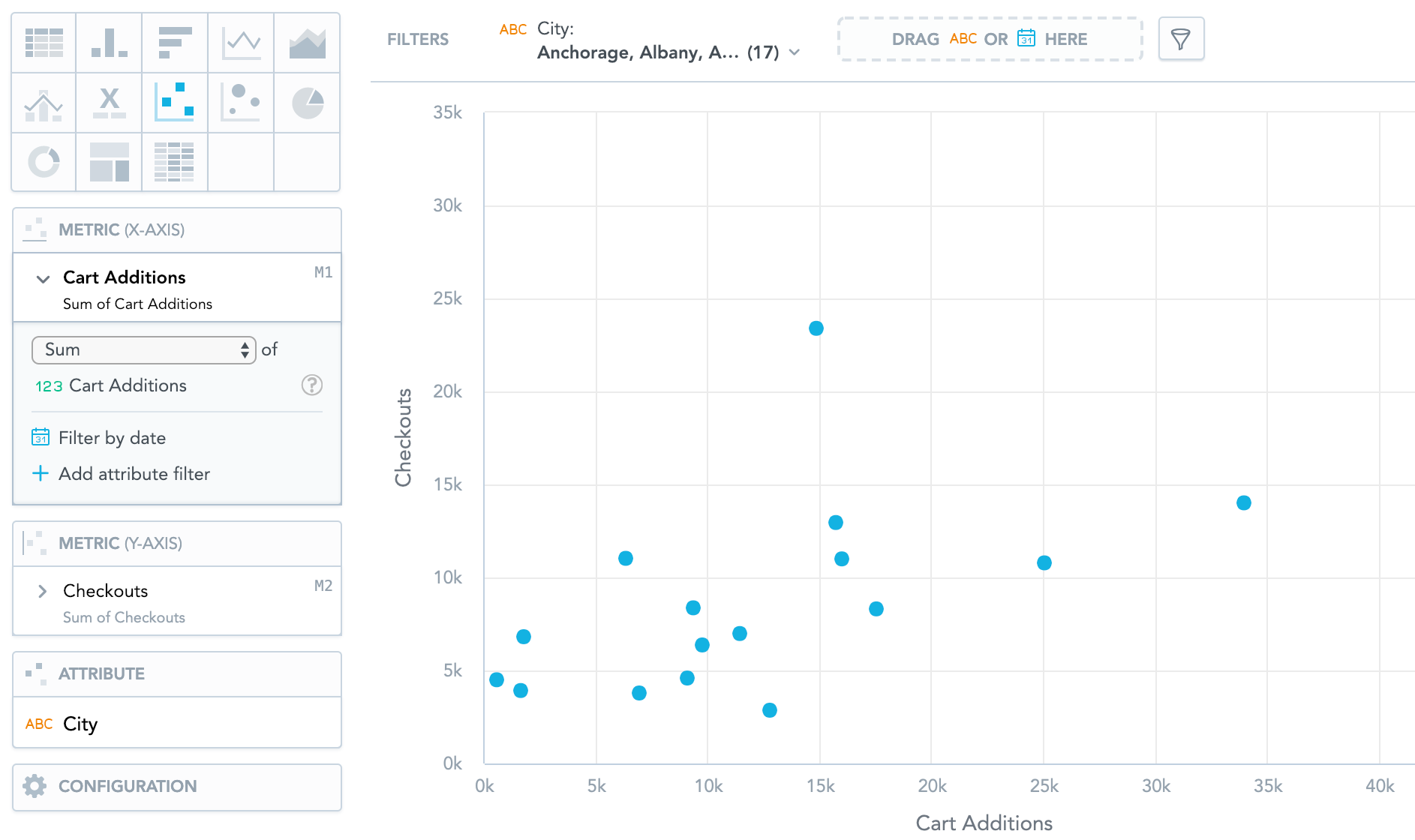# Scatter Plots

Scatter plots show data as points using Cartesian coordinates. Scatter plots can have two metrics, one for the X-axis and the other for the Y-axis, and one attribute, which determines the meaning of each data point. You can use scatter plots to analyze trends between two metrics or to track the magnitude of two metrics from the same chart.

Scatter plots have the following sections: Metric (X-axis), Metric (Y-axis), Attribute, and Configuration.

For information about common characteristics and settings of all insights, see Insight Types.